In this lab, we will cover some state-of-the-art techniques in the framework of tree models. We use the same datasets as in previous lab, Boston Housing data and Credit Scoring data.

# load Boston data
library(MASS)
data(Boston)
index <- sample(nrow(Boston),nrow(Boston)*0.9)
boston_train <- Boston[index,]
boston_test <- Boston[-index,]

# 1 Random Forests

Random forest is an extension of Bagging, but it makes significant improvement in terms of prediction. The idea of random forests is to randomly select $$m$$ out of $$p$$ predictors as candidate variables for each split in each tree. Commonly, $$m=\sqrt{p}$$. The reason of doing this is that it can decorrelates the trees such that it reduces variance when we aggregate the trees. You may refer Wikipedia and the tutorial on the author’s website. Note: The current randomForest package do not handle asymmetric loss.

## 1.1 Random Forest for Regression

library(randomForest)
boston_rf<- randomForest(medv~., data = boston_train, importance=TRUE)
boston_rf
##
## Call:
##  randomForest(formula = medv ~ ., data = boston_train, importance = TRUE)
##                Type of random forest: regression
##                      Number of trees: 500
## No. of variables tried at each split: 4
##
##           Mean of squared residuals: 10.56731
##                     % Var explained: 87.26
mod_rf <- randomForest(medv~., data=boston_train,
importance=TRUE, ntree=500)

By default, $$m=p/3$$ for regression tree, and $$m=\sqrt{p}$$ for classification problem. You can change it by specifying mtry=. You can also specify number of trees by ntree=. The default is 500. The argument importance=TRUE allows us to see the variable imporatance.

boston_rf$importance ## %IncMSE IncNodePurity ## crim 7.4891565 2133.3077 ## zn 1.0150814 322.1778 ## indus 6.8103051 2595.3081 ## chas 0.7690971 301.1793 ## nox 8.3532893 1859.9877 ## rm 36.8017260 11708.3008 ## age 3.5398249 1061.4449 ## dis 5.6934693 1791.6105 ## rad 1.1223798 301.7534 ## tax 4.0933430 1405.2961 ## ptratio 7.4723517 2610.2498 ## black 1.4149655 666.1111 ## lstat 47.6952832 10073.5542 mod_rf$importance
##            %IncMSE IncNodePurity
## crim     7.5154868     2211.2678
## zn       0.5709418      243.6042
## indus    7.3905105     2707.8133
## chas     1.1367065      344.2968
## nox      9.1721474     2320.7097
## rm      35.0694205    11112.5503
## age      3.5510146     1061.8914
## dis      5.3369928     2040.2222
## tax      3.6163376     1261.4381
## ptratio  6.8784967     2594.7292
## black    1.5956818      706.3922
## lstat   46.6112346    10317.9420

The MSR is MSE of out-of-bag prediction (recall the OOB in bagging). The fitted randomForest actually saves all OOB errors for each ntree value from 1 to 500. We can make a plot to see how the OOB error changes with different ntree.

plot(boston_rf$mse, type='l', col=2, lwd=2, xlab = "ntree", ylab = "OOB Error")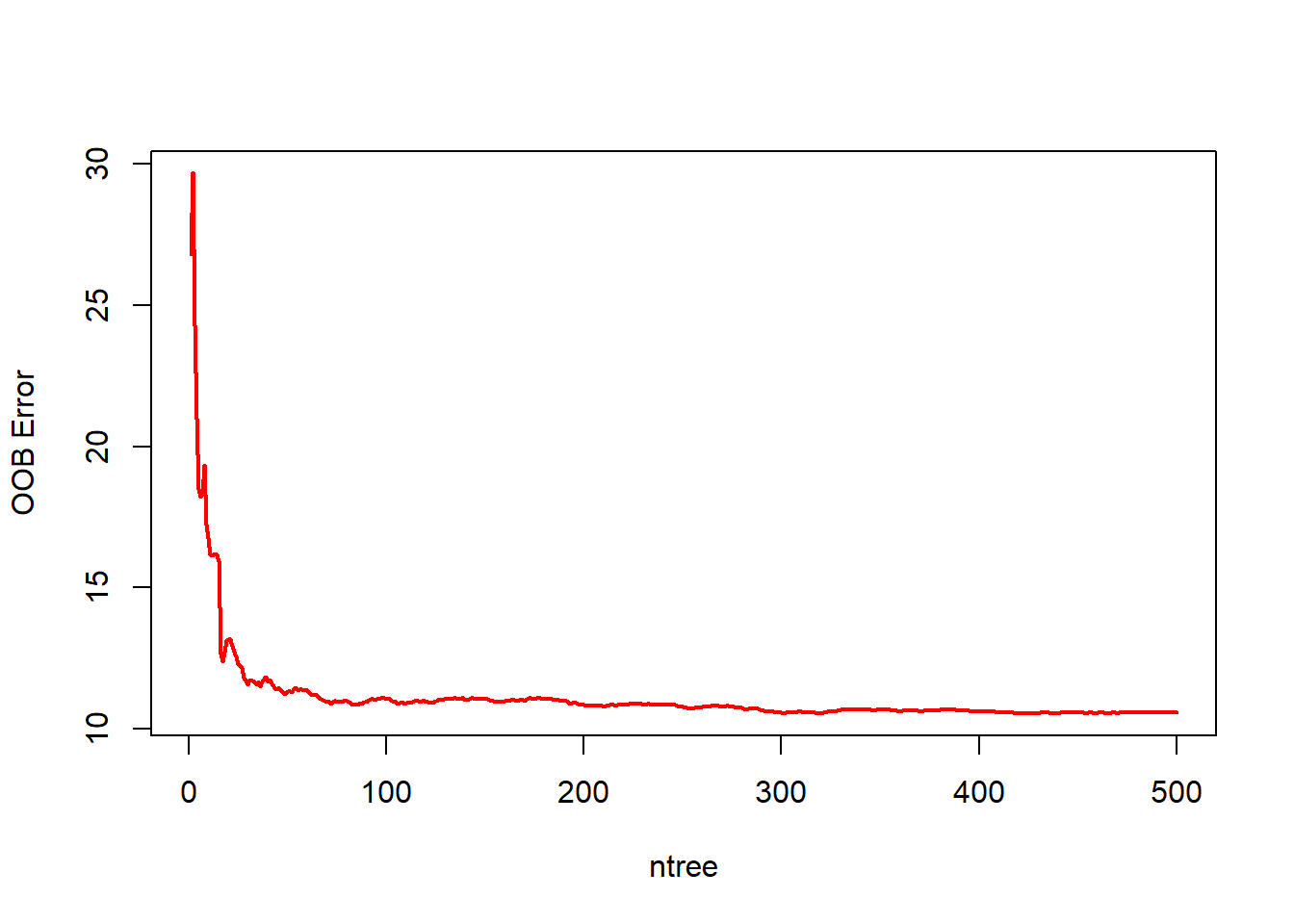plot(mod_rf$mse, type='l', col=2, lwd=2, xlab = "ntree", ylab = "OOB Error")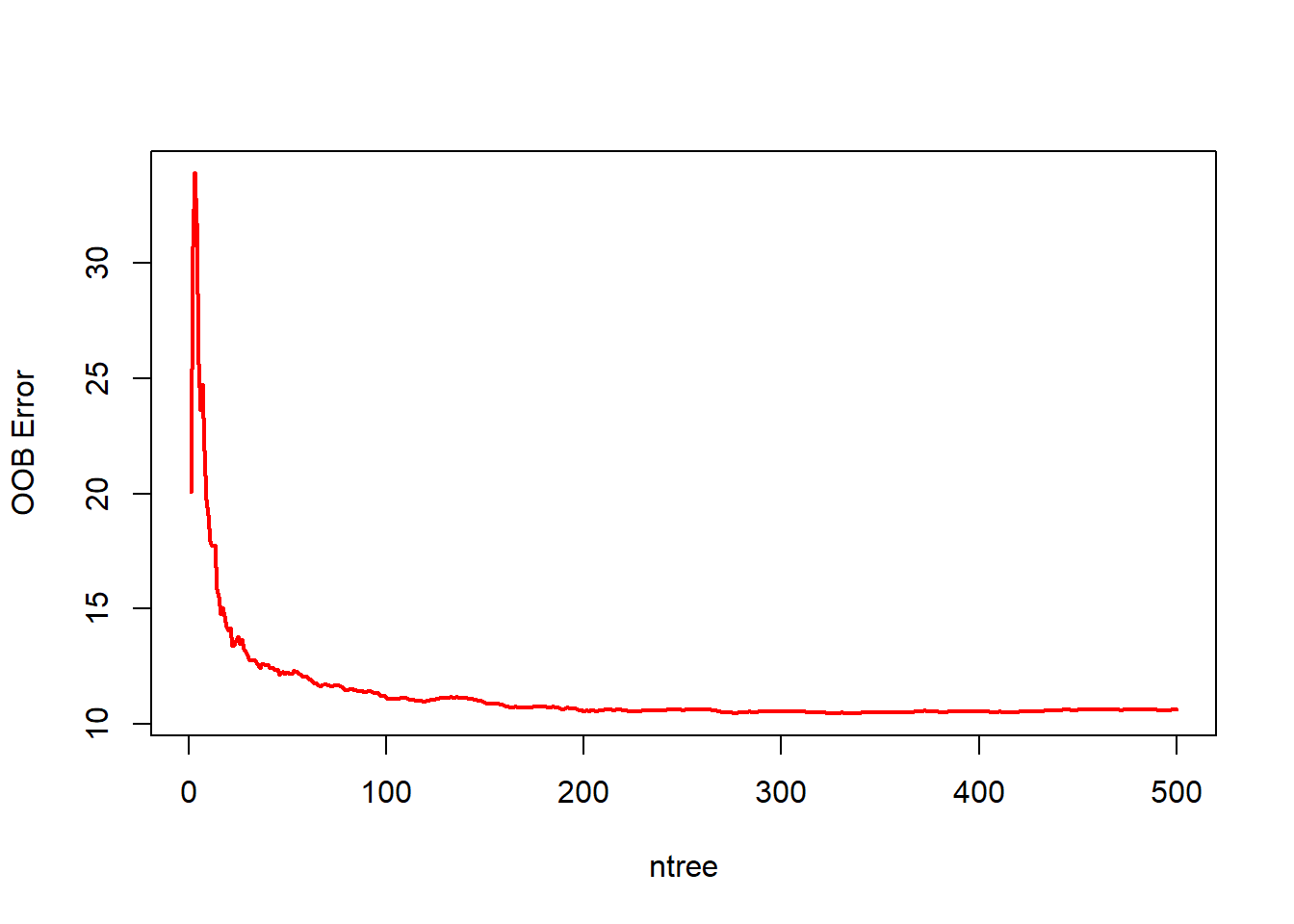Prediction on the testing sample.

boston_rf_pred<- predict(boston_rf, boston_test)
mean((boston_test$medv-boston_rf_pred)^2) ##  19.35997 As we mentioned before, the number of candidate predictors in each split is $$m=p/3\approx 4$$. We can also specify $$m$$ with argument mtry. Now let’s see how the OOB error and testing error changes with mtry. oob_err <- rep(0, 13) test.err <- rep(0, 13) for(i in 1:13){ fit<- randomForest(medv~., data = boston_train, mtry=i) oob_err[i]<- fit$mse
test.err[i]<- mean((boston_test$medv-predict(fit, boston_test))^2) cat(i, " ") } ## 1 2 3 4 5 6 7 8 9 10 11 12 13 matplot(cbind(test.err, oob_err), pch=15, col = c("red", "blue"), type = "b", ylab = "MSE", xlab = "mtry") legend("topright", legend = c("test Error", "OOB Error"), pch = 15, col = c("red", "blue"))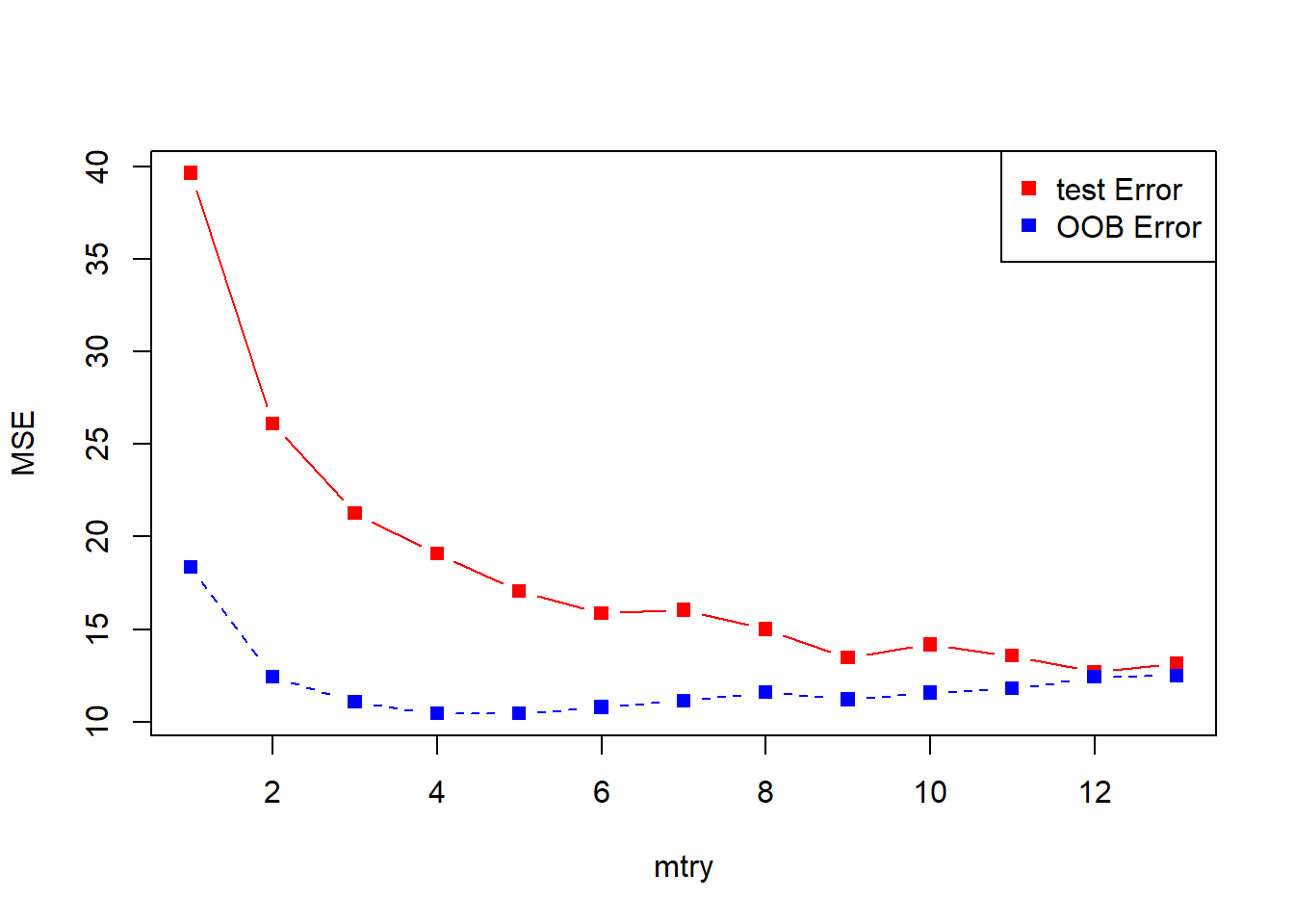Exercise: Create a plot displaying the test error across ntree=1, …, 500, and mtry= 1, …, 13. (You can draw 13 lines in different color representing each $$m$$). ## 1.2 Random Forest for Classification We apply the random forest model to the credit card dataset: # load credit card data credit_data <- read.csv(file = "https://xiaoruizhu.github.io/Data-Mining-R/lecture/data/credit_default.csv", header=T) # convert categorical variables credit_data$SEX<- as.factor(credit_data$SEX) credit_data$EDUCATION<- as.factor(credit_data$EDUCATION) credit_data$MARRIAGE<- as.factor(credit_data$MARRIAGE) # random splitting index <- sample(nrow(credit_data),nrow(credit_data)*0.9) credit_train = credit_data[index,] credit_test = credit_data[-index,] credit_rf <- randomForest(as.factor(default.payment.next.month)~., data = credit_train, importance=TRUE, ntree=500) credit_rf ## ## Call: ## randomForest(formula = as.factor(default.payment.next.month) ~ ., data = credit_train, importance = TRUE, ntree = 500) ## Type of random forest: classification ## Number of trees: 500 ## No. of variables tried at each split: 4 ## ## OOB estimate of error rate: 18.43% ## Confusion matrix: ## 0 1 class.error ## 0 7897 489 0.05831147 ## 1 1501 913 0.62178956 We can again easily plot the error rate vs. ntree. However, as far as I know, randomForest does not support asymmetric loss either. So it always uses the overall misclassification rate as the error. plot(credit_rf, lwd=rep(2, 3)) legend("right", legend = c("OOB Error", "FPR", "FNR"), lwd=rep(2, 3), lty = c(1,2,3), col = c("black", "red", "green"))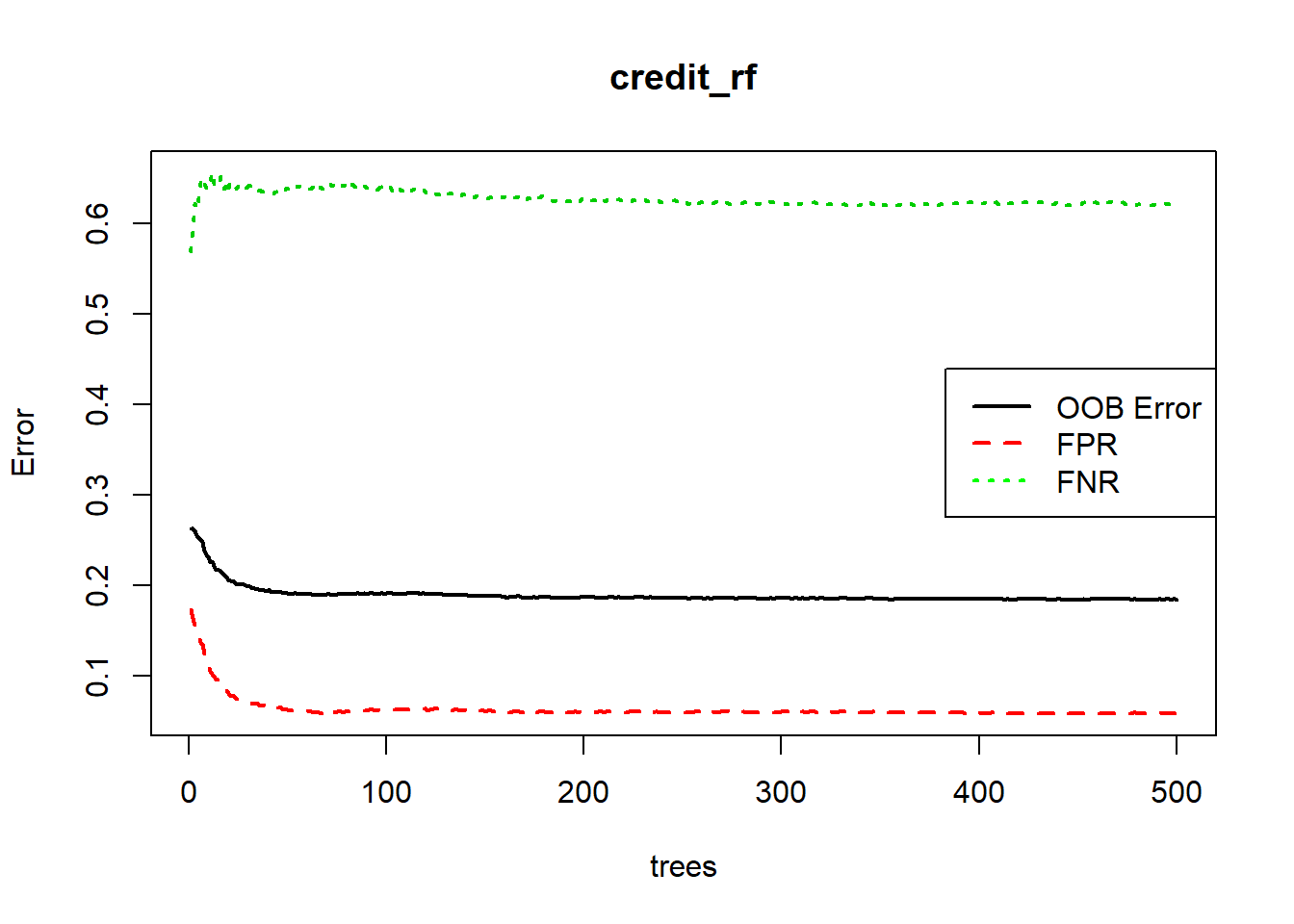ROC curve and AUC can be obtained based on the probability prediction. credit_rf_pred <- predict(credit_rf, type = "prob")[,2] library(ROCR) pred <- prediction(credit_rf_pred, credit_train$default.payment.next.month)
perf <- performance(pred, "tpr", "fpr")
plot(perf, colorize=TRUE)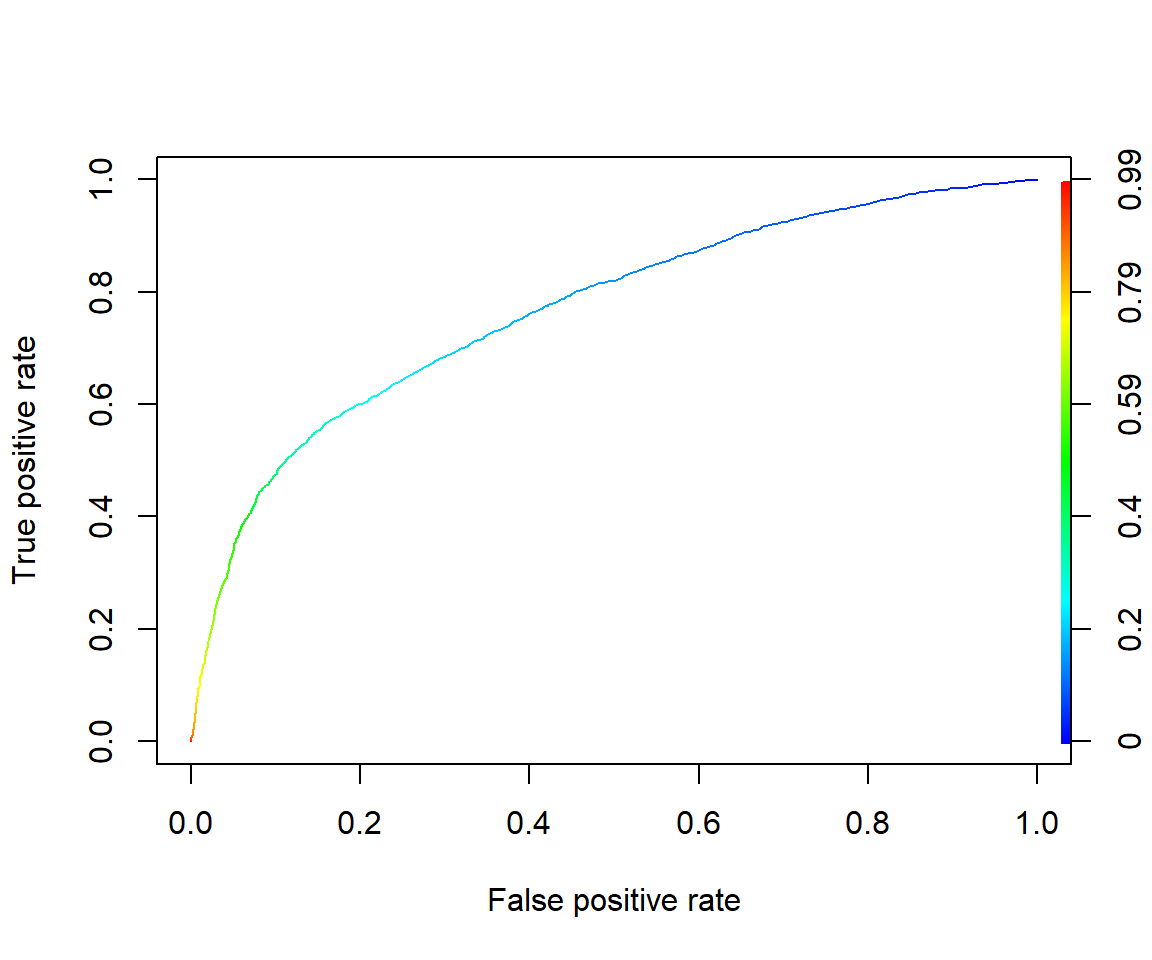#Get the AUC
unlist(slot(performance(pred, "auc"), "y.values"))
##  0.7671521

Create the confusion matrix based on the cutoff probability from asymmetric cost (pcut=1/6).

## out-of-sample
pcut <- 1/6
credit_rf_pred_test <- predict(credit_rf, newdata=credit_test, type = "prob")[,2]
credit_rf_class_test <- (credit_rf_pred_test>pcut)*1
table(credit_test\$default.payment.next.month, credit_rf_class_test, dnn = c("True", "Pred"))
##     Pred
## True   0   1
##    0 588 394
##    1  57 161